In :
# ignore next line, only necessary for plotting images in HTML through iPython notebook

In :
%matplotlib inline


# Plot netCDF data on a map¶

First we need to import netCDF4-python, Numpy, Matplotlib for plotting, and Basemap for the map

In :
from netCDF4 import Dataset as NetCDFFile
import matplotlib.pyplot as plt
import numpy as np
from mpl_toolkits.basemap import Basemap


In :
nc = NetCDFFile('/Users/corymartin/Downloads/ECMWF_ERA-40_subset.nc') # note this file is 2.5 degree, so low resolution data


Read the variables from the netCDF file and assign them to Python variables

In :
lat = nc.variables['latitude'][:]
lon = nc.variables['longitude'][:]
time = nc.variables['time'][:]
t2 = nc.variables['p2t'][:] # 2 meter temperature
mslp = nc.variables['msl'][:] # mean sea level pressure
u = nc.variables['p10u'][:] # 10m u-component of winds
v = nc.variables['p10v'][:] # 10m v-component of winds


Let's create a map centered over Columbus, OH (40N,83W) and extending 20 degrees W-E and 10 degrees N-S

In :
map = Basemap(projection='merc',llcrnrlon=-93.,llcrnrlat=35.,urcrnrlon=-73.,urcrnrlat=45.,resolution='i') # projection, lat/lon extents and resolution of polygons to draw
# resolutions: c - crude, l - low, i - intermediate, h - high, f - full


Now let's add the states, counties, coasts, national borders and a land-sea colored mask:

In :
map.drawcoastlines()
map.drawstates()
map.drawcountries()
map.drawlsmask(land_color='Linen', ocean_color='#CCFFFF') # can use HTML names or codes for colors
map.drawcounties() # you can even add counties (and other shapefiles!)

Out:
<matplotlib.collections.LineCollection at 0x106966fd0>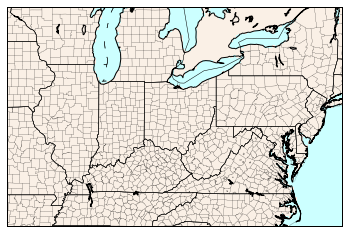If you'd like to add Lat/Lon, you can do that too:

In :
parallels = np.arange(30,50,5.) # make latitude lines ever 5 degrees from 30N-50N
meridians = np.arange(-95,-70,5.) # make longitude lines every 5 degrees from 95W to 70W
map.drawparallels(parallels,labels=[1,0,0,0],fontsize=10)
map.drawmeridians(meridians,labels=[0,0,0,1],fontsize=10)

Out:
{-90.0: ([<matplotlib.lines.Line2D at 0x10e485750>],
[<matplotlib.text.Text at 0x10e4a52d0>]),
-85.0: ([<matplotlib.lines.Line2D at 0x10e485890>],
[<matplotlib.text.Text at 0x10e4a57d0>]),
-80.0: ([<matplotlib.lines.Line2D at 0x10e4927d0>],
[<matplotlib.text.Text at 0x10e4a5c10>]),
-75.0: ([<matplotlib.lines.Line2D at 0x10e492d90>],
[<matplotlib.text.Text at 0x10e4b0090>])}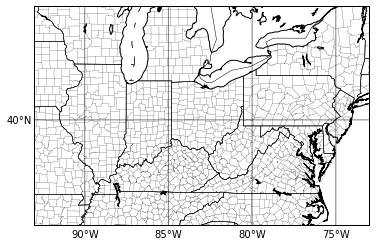Now, let's prepare the data for the map.

We have to transform the lat/lon data to map coordinates.

In :
lons,lats= np.meshgrid(lon-180,lat) # for this dataset, longitude is 0 through 360, so you need to subtract 180 to properly display on map
x,y = map(lons,lats)


After that, we can then plot the netCDF data on the map.

In :
clevs = np.arange(960,1040,4)
cs = map.contour(x,y,mslp[0,:,:]/100.,clevs,colors='blue',linewidths=1.)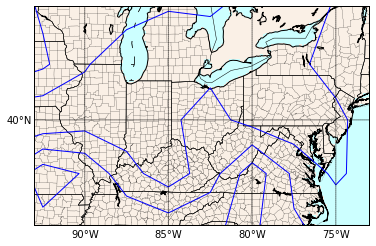In :
plt.clabel(cs, fontsize=9, inline=1) # contour labels
plt.title('Mean Sea Level Pressure')

Out:
<matplotlib.text.Text at 0x11dcdc910>

/Users/corymartin/anaconda/lib/python2.7/site-packages/matplotlib/text.py:52: UnicodeWarning: Unicode equal comparison failed to convert both arguments to Unicode - interpreting them as being unequal
if rotation in ('horizontal', None):
/Users/corymartin/anaconda/lib/python2.7/site-packages/matplotlib/text.py:54: UnicodeWarning: Unicode equal comparison failed to convert both arguments to Unicode - interpreting them as being unequal
elif rotation == 'vertical':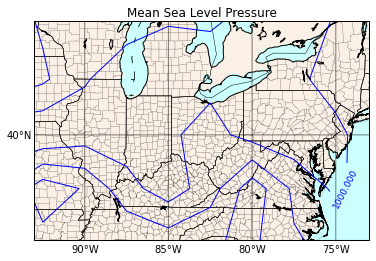If you'd rather plot temperature, we can do that too (and with a colorbar).

In :
temp = map.contourf(x,y,t2[4,:,:])
plt.title('2m Temperature')
cb.set_label('Temperature (K)')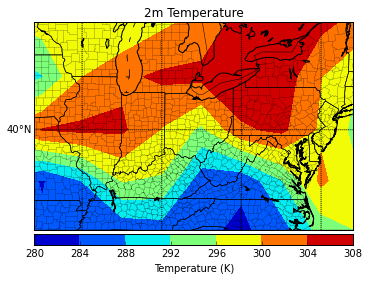Don't forget to show or save the figure!

(note if you want to create plots in an automated script (aka without X-Window at all), add the following to the very top of your script:

import matplotlib matplotlib.use('Agg')

This will allow matplotlib to use the Agg backend instead of Qt, and will create plots in a batch format, rather than interactively.

In :
plt.show()
plt.savefig('2m_temp.png')

<matplotlib.figure.Figure at 0x106991b90>


Now you've seen the basics on how to plot netCDF data in Python! The power of Matplotlib allows you to make these plots beautiful easily, with annotated points, dozens of colorbars to choose from, and LaTeX for writing mathematical formulas in titles/labels/annotations.

Back to Examples